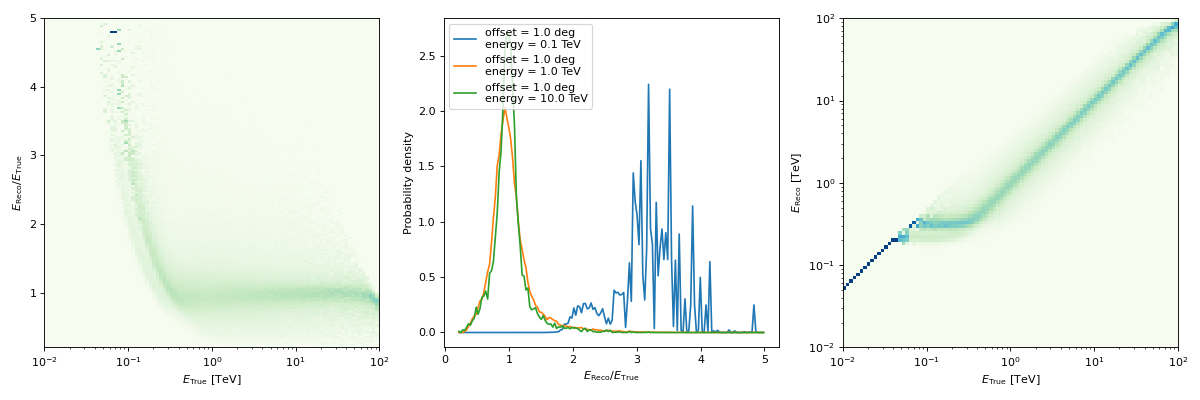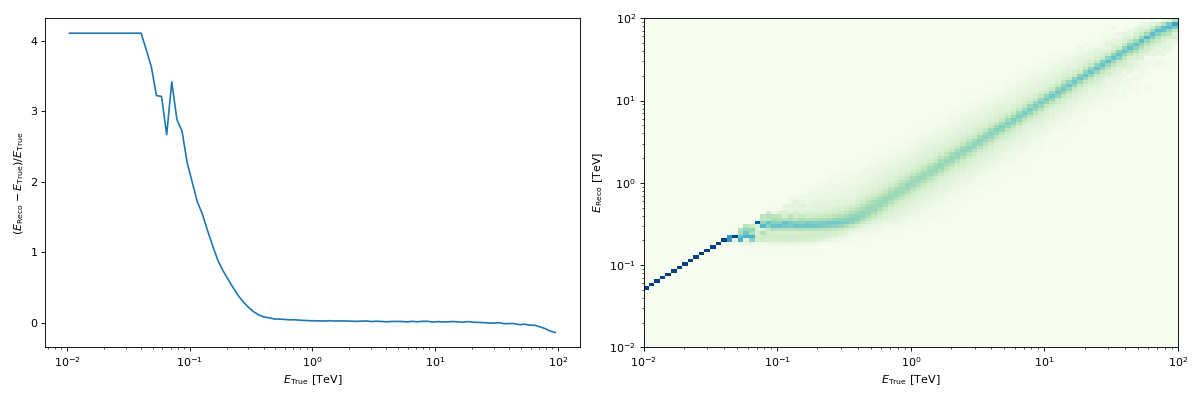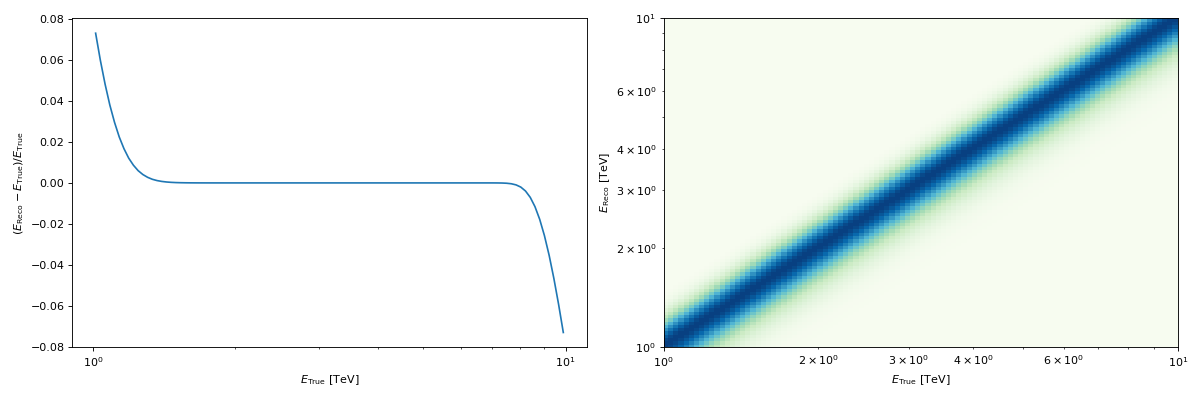# Energy Dispersion¶

## as a function of of true energy and offset angle (EDISP_2D)¶

The EnergyDispersion2D class represents the probability density of the energy migration $$\mu=\frac{E}{E_{\rm true}}$$ as a function of true energy and offset angle from the field of view center ($$E_{\rm disp}(E_{\rm true}, \mu|p_{\rm true})$$ in IRF Theory).

Its format specifications are available in EDISP_2D

This is the format in which IACT DL3 energy dispersions are usually provided, as an example:

"""Plot an energy dispersion from the HESS DL3 data release 1."""
import matplotlib.pyplot as plt
from gammapy.irf import EnergyDispersion2D

filename = "$GAMMAPY_DATA/hess-dl3-dr1/data/hess_dl3_dr1_obs_id_020136.fits.gz" edisp = EnergyDispersion2D.read(filename, hdu="EDISP") edisp.peek() plt.show()  (png, hires.png, pdf)## as a function of true energy (RMF)¶ EDispKernel instead represents an energy dispersion as a function of true energy only ($$E_{\rm disp}(E| E_{\rm true})$$ following the notation in IRF Theory). EDispKernel contains the energy redistribution matrix (or redistribution matrix function, RMF, in the OGIP standard). The energy redistribution provides the integral of the energy dispersion probability function over bins of reconstructed energy. It is used to convert vectors of predicted counts in true energy in vectors of predicted counts in reconstructed energy. Its format specifications are available in RMF. Such an energy dispersion can be obtained for example: """Plot the energy dispersion at a given offset.""" from astropy.coordinates import Angle import matplotlib.pyplot as plt from gammapy.irf import EnergyDispersion2D filename = "$GAMMAPY_DATA/hess-dl3-dr1/data/hess_dl3_dr1_obs_id_020136.fits.gz"
# offset at which we want to examine the energy dispersion
offset = Angle("0.5 deg")
edisp_kernel = edisp.to_edisp_kernel(offset=offset)
edisp_kernel.peek()
plt.show()


(png, hires.png, pdf)• or starting from a parameterisation:

"""Plot an energy dispersion using a gaussian parametrisation"""
import numpy as np
import astropy.units as u
import matplotlib.pyplot as plt
from gammapy.irf import EDispKernel

energy = np.logspace(0, 1, 101) * u.TeV
edisp = EDispKernel.from_gauss(energy=energy, energy_true=energy, sigma=0.1, bias=0,)
edisp.peek()
plt.show()


(png, hires.png, pdf)